Function Repository Resource:

# PopulationStandardDeviation

Compute the population standard deviation for a set of data

Contributed by: Wolfram|Alpha Math Team
 ResourceFunction["PopulationStandardDeviation"][{x1,x2,…}] gives the population standard deviation of the given data.

## Details

ResourceFunction["PopulationStandardDeviation"][] is equivalent to Sqrt[ResourceFunction["PopulationVariance"][]].
ResourceFunction["PopulationStandardDeviation"] handles both numerical and symbolic data.

## Examples

### Basic Examples (3)

Compute the population standard deviation of a list:

 In:=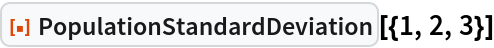Out=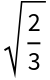Compute the population standard deviation of another list:

 In:=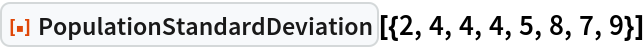Out=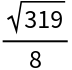Compute the population standard deviation of another list:

 In:=Out=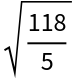Compare with the known expression for population standard deviation as the root-mean-square difference to the mean:

 In:=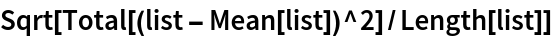Out=### Scope (1)

PopulationStandardDeviation works on both numeric and symbolic input:

 In:=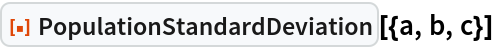Out=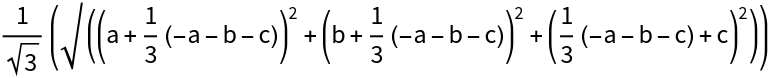### Properties and Relations (2)

The population standard deviation of a dataset differs from its sample standard deviation calculated with StandardDeviation due to Bessel's correction:

 In:=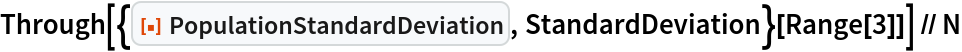Out=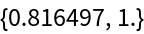The fractional effect of this correction tends to be smaller for larger sample sizes:

 In:=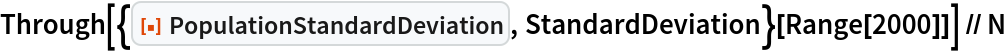Out=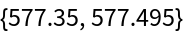PopulationStandardDeviation[]is equivalent to Sqrt[ResourceFunction["PopulationVariance"][]]:

 In:=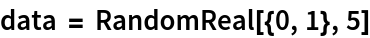Out=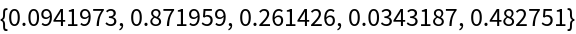In:=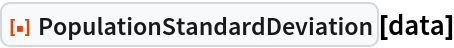Out=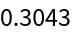In:=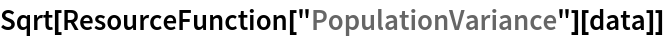Out=## Publisher

Wolfram|Alpha Math Team

## Version History

• 4.0.0 – 23 March 2023
• 3.1.0 – 11 May 2021
• 3.0.0 – 24 January 2020
• 2.0.0 – 06 September 2019
• 1.0.0 – 05 August 2019